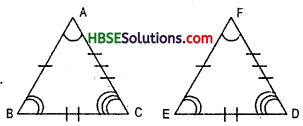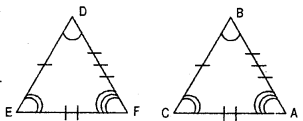# HBSE 7th Class Maths Solutions Chapter 7 Congruence of Triangles Ex 7.1

Haryana State Board HBSE 7th Class Maths Solutions Chapter 7 Congruence of Triangles Ex 7.1 Textbook Exercise Questions and Answers.

## Haryana Board 7th Class Maths Solutions Chapter 7 Congruence of Triangles Exercise 7.1

Question 1.
Complete the following statements:
(a) Two line segments are congruent if
(b) Among two congruent angles one has a measure 70°; the measure of the other angle is .
(c) When we write ∠A ≅ ∠B we actually mean
Solution:
(a) their length are same.
(b) 70° (c) ∠A ≅ ∠B.

Question 2.
Give any two real-life examples for congruent shapes.
Solution:
(i) Ears
(ii) Eyes

Question 3.
If ∠ABC = ∠FED under the correspondence ABC ⇔ FED, write all the corresponding congruent parts of the triangles.Solution:
If ΔABC ≅ ΔFED
then AB = FE
BC = ED
AC = FD
and ∠A = ∠F,
∠B =∠E and ∠C = ∠D.Question 4.
If ΔDEF ≅ ΔBCA, write the part (s) of ABCA that correspond to
(i) ∠E
(ii) $$\overline{E F}$$
(iii) ∠F
(iv) $$\overline{D F}$$(i) ∠E ⇔ ∠F
(ii) $$\overline{E F}$$ ⇔ $$\overline{C A}$$
(iii) ∠F ⇔∠A
(iv) $$\overline{D F}$$ ⇔ $$\overline{B A}$$